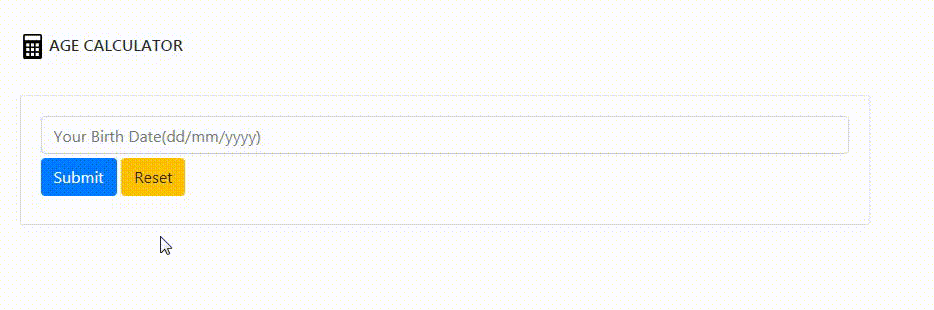Related Articles

# How to create AGE Calculator Web App PyWebIO in Python ?

• Difficulty Level : Medium
• Last Updated : 17 Jun, 2021

In this article, we are going to create an AGE Calculator that will show your age in years, months, and days with the help of the current date. We will use the PyWebIO module for creating a simple and interactive interface on the web. This is a python module mostly used to create simple and interactive interfaces on the web using Python programming. It can be installed using the below command:

`pip install pywebio`

Stepwise Implementation:

Step 1: Import all the required modules.

## Python3

 `# Import the following modules``from` `dateutil.relativedelta ``import` `relativedelta``from` `datetime ``import` `datetime``from` `time ``import` `strptime``from` `pywebio.``input` `import` `*``from` `pywebio.output ``import` `*``from` `pywebio.session ``import` `*``import` `time`

Step 2: Getting current time and taking input from the user.

## Python3

 `# Getting Current time.``date ``=` `datetime.now().strftime(``"%d/%m/%Y"``)  `` ` `# Taking age from the user``DOB ``=` `input``("``", placeholder = "``Your Birth Date(dd``/``mm``/``yyyy)")`

Step 3: Checking whether the format of age is correct or not.

## Python3

 `try``:``    ``# Check whether the input age format ``    ``# is same as given format``    ``val ``=` `strptime(DOB, ``"%d/%m/%Y"``)``except``:``   ` `    ``# If format is different, then through ``    ``# an error.``    ``put_error(``"Alert! This is not the right format"``)``    ``time.sleep(``3``)  ``# sleep for 3 seconds``    ``continue`

Step 4: Split the Birth Date of the user and the Current Date by ‘/’. And then Typecast all the split parts into the integer. Swap months and years for both the user’s birth date and current date.

## Python3

 `# Split the age by '/'``in_date ``=` `DOB.split(``'/'``)`` ` `# split the todays date by '/'``date ``=` `date.split(``'/'``)  `` ` `# Typecast all the converted part ``# into the int.``in_date ``=` `[``int``(i) ``for` `i ``in` `in_date] ``date ``=` `[``int``(i) ``for` `i ``in` `date] ``newdate ``=` `[]  `` ` `# Swap days with years``in_date[``0``], in_date[``2``] ``=` `in_date[``2``], in_date[``0``]  `` ` `# Swap days with years``date[``0``], date[``2``] ``=` `date[``2``], date[``0``]`

Step 5: Check whether or not the current year is smaller than the User’s D.O.B year. If the current year is smaller than through an error.

## Python3

 `if` `in_date <``=` `date:``        ``now ``=` `datetime.strptime(DOB, ``"%d/%m/%Y"``)``     ` `        ``# Display output in a pop window``        ``popup(``"Your Age"``,k``              ``[put_html(``"

"``f"{relativedelta(datetime.now(),now).years} Years<``/``br> \``              ``{relativedelta(datetime.now(),now).months} Months<``/``br>\``              ``{relativedelta(datetime.now(),now).days} Days"``"

"``), put_buttons(``                  ``[``'Close'``], onclick``=``lambda` `_: close_popup())], implicit_close``=``True``)``else``:``        ``# If you input the year greater than current year``        ``put_warning(``            ``f``"No result found, this is {date}, and you can't be in {in_date}."``)`

Complete Code:

## Python3

 `# Import the following modules``from` `dateutil.relativedelta ``import` `relativedelta``from` `datetime ``import` `datetime``from` `time ``import` `strptime``from` `pywebio.``input` `import` `*``from` `pywebio.output ``import` `*``from` `pywebio.session ``import` `*``import` `time`` ` `# Run infinite loop``while` `True``:  ``    ``clear() ``     ` `    ``# Put a heading Age Calculator``    ``put_html(``"

AGE CALCULATOR<``/``h4><``/``p>``")``     ` `    ``# Getting Current time.``    ``date ``=` `datetime.now().strftime(``"%d/%m/%Y"``)  ``     ` `    ``# Taking age from the user``    ``DOB ``=` `input``("``", placeholder="``Your Birth Date(dd``/``mm``/``yyyy)")``    ``try``:``       ` `        ``# Check whether the input age``        ``# format is same as given format``        ``val ``=` `strptime(DOB, ``"%d/%m/%Y"``)``    ``except``:``       ` `        ``# If format is different, then through an error.``        ``put_error(``"Alert! This is not the right format"``)``         ` `        ``# sleep for 3 seconds``        ``time.sleep(``3``)  ``        ``continue``    ``in_date ``=` `DOB.split(``'/'``) ``    ``date ``=` `date.split(``'/'``)  ``     ` `    ``# Typecast all the converted part into the int.``    ``in_date ``=` `[``int``(i) ``for` `i ``in` `in_date]``    ``date ``=` `[``int``(i) ``for` `i ``in` `date]``     ` `    ``# Define an empty list``    ``newdate ``=` `[]  ``     ` `    ``# Swap days with years``    ``in_date[``0``], in_date[``2``] ``=` `in_date[``2``], in_date[``0``]  ``     ` `    ``# Swap days with years``    ``date[``0``], date[``2``] ``=` `date[``2``], date[``0``]  ``    ``if` `in_date <``=` `date:``        ``now ``=` `datetime.strptime(DOB, ``"%d/%m/%Y"``)``         ` `        ``# Display output``        ``popup(``"Your Age"``,``              ``[put_html(``"

"``f"{relativedelta(datetime.now(),now).years} Years<``/``br> \``              ``{relativedelta(datetime.now(),now).months} Months<``/``br>\``              ``{relativedelta(datetime.now(),now).days} Days"``"

"``), put_buttons(``                  ``[``'Close'``], onclick``=``lambda` `_: close_popup())], implicit_close``=``True``)``    ``else``:``       ` `        ``# If you input the year greater than current year``        ``put_warning(``            ``f``"No result found, this is {date}, and you can't be in {in_date}."``)``        ``time.sleep(``3``)``    ``clear()``    ``# Give user a choice``    ``choice ``=` `radio(``"Do you want to calculate again?"``,``                   ``options``=``[``'Yes'``, ``'No'``], required``=``True``)``    ``if` `choice.lower() ``=``=` `'yes'``:``        ``continue``    ``else``:``        ``clear()``         ` `        ``# Show a toast notification``        ``toast(``"Thanks a lot!"``)  ``        ``exit()`

Output:Attention geek! Strengthen your foundations with the Python Programming Foundation Course and learn the basics.

To begin with, your interview preparations Enhance your Data Structures concepts with the Python DS Course. And to begin with your Machine Learning Journey, join the Machine Learning – Basic Level Course

My Personal Notes arrow_drop_up